# Multivariate quantiles and long horizon forecasting with N-HiTS#

:

import os
import warnings

warnings.filterwarnings("ignore")

os.chdir("../../..")

:

import matplotlib.pyplot as plt
import numpy as np
import pandas as pd
import pytorch_lightning as pl
from pytorch_lightning.callbacks import EarlyStopping
import torch

from pytorch_forecasting import Baseline, NHiTS, TimeSeriesDataSet
from pytorch_forecasting.data import NaNLabelEncoder
from pytorch_forecasting.data.examples import generate_ar_data
from pytorch_forecasting.metrics import SMAPE, MQF2DistributionLoss, QuantileLoss


We generate a synthetic dataset to demonstrate the network’s capabilities. The data consists of a quadratic trend and a seasonality component.

:

data = generate_ar_data(seasonality=10.0, timesteps=400, n_series=100, seed=42)
data["static"] = 2
data["date"] = pd.Timestamp("2020-01-01") + pd.to_timedelta(data.time_idx, "D")

:

series time_idx value static date
0 0 0 -0.000000 2 2020-01-01
1 0 1 -0.046501 2 2020-01-02
2 0 2 -0.097796 2 2020-01-03
3 0 3 -0.144397 2 2020-01-04
4 0 4 -0.177954 2 2020-01-05

Before starting training, we need to split the dataset into a training and validation TimeSeriesDataSet.

:

# create dataset and dataloaders
max_encoder_length = 60
max_prediction_length = 20

training_cutoff = data["time_idx"].max() - max_prediction_length

context_length = max_encoder_length
prediction_length = max_prediction_length

training = TimeSeriesDataSet(
data[lambda x: x.time_idx <= training_cutoff],
time_idx="time_idx",
target="value",
categorical_encoders={"series": NaNLabelEncoder().fit(data.series)},
group_ids=["series"],
# only unknown variable is "value" - and N-HiTS can also not take any additional variables
time_varying_unknown_reals=["value"],
max_encoder_length=context_length,
max_prediction_length=prediction_length,
)

validation = TimeSeriesDataSet.from_dataset(training, data, min_prediction_idx=training_cutoff + 1)
batch_size = 128


## Calculate baseline error#

Our baseline model predicts future values by repeating the last know value. The resulting SMAPE is disappointing and should not be easy to beat.

:

# calculate baseline absolute error
actuals = torch.cat([y for x, y in iter(val_dataloader)])
SMAPE()(baseline_predictions, actuals)

:

tensor(0.5462)


## Train network#

Finding the optimal learning rate using [PyTorch Lightning](https://pytorch-lightning.readthedocs.io/) is easy. The key hyperparameter of the NHiTS model is hidden_size.

PyTorch Forecasting is flexible enough to use NHiTS with different loss functions, enabling not only point forecasts but also probabilistic ones. Here, we will demonstrate not only a typical quantile regression but a multivariate quantile regression with MQF2DistributionLoss which allows calculation sampling consistent paths along a prediction horizon. This allows calculation of quantiles, e.g. of the sum over the prediction horizon while still avoiding the accumulating error problem from auto-regressive methods such as DeepAR. One needs to install an additional package for this quantile function:

pip install pytorch-forecasting[mqf2]

:

pl.seed_everything(42)
net = NHiTS.from_dataset(
training,
learning_rate=3e-2,
weight_decay=1e-2,
loss=MQF2DistributionLoss(prediction_length=max_prediction_length),
backcast_loss_ratio=0.0,
hidden_size=64,
)

Global seed set to 42
GPU available: False, used: False
TPU available: False, using: 0 TPU cores
IPU available: False, using: 0 IPUs
HPU available: False, using: 0 HPUs

:

# find optimal learning rate
print(f"suggested learning rate: {res.suggestion()}")
fig = res.plot(show=True, suggest=True)
fig.show()
net.hparams.learning_rate = res.suggestion()

Restoring states from the checkpoint path at /Users/beitnerjan/Documents/Github/temporal_fusion_transformer_pytorch/.lr_find_147670cc-2986-41d7-b05a-ae03fe545b02.ckpt

suggested learning rate: 0.08912509381337459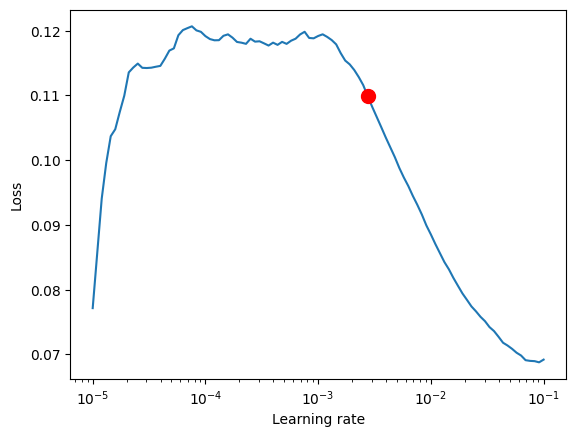Fit model

:

early_stop_callback = EarlyStopping(monitor="val_loss", min_delta=1e-4, patience=10, verbose=False, mode="min")
trainer = pl.Trainer(
max_epochs=5,
gpus=0,
enable_model_summary=True,
callbacks=[early_stop_callback],
limit_train_batches=30,
enable_checkpointing=True,
)

net = NHiTS.from_dataset(
training,
learning_rate=0.09,
log_interval=10,
log_val_interval=1,
weight_decay=1e-2,
backcast_loss_ratio=0.0,
hidden_size=64,
loss=MQF2DistributionLoss(prediction_length=max_prediction_length),
)

trainer.fit(
net,
)

GPU available: False, used: False
TPU available: False, using: 0 TPU cores
IPU available: False, using: 0 IPUs
HPU available: False, using: 0 HPUs

| Name            | Type                 | Params
---------------------------------------------------------
0 | loss            | MQF2DistributionLoss | 5.1 K
1 | logging_metrics | ModuleList           | 0
2 | embeddings      | MultiEmbedding       | 0
3 | model           | NHiTS                | 35.7 K
---------------------------------------------------------
40.8 K    Trainable params
0         Non-trainable params
40.8 K    Total params
0.163     Total estimated model params size (MB)


### Evaluate Results#

:

best_model_path = trainer.checkpoint_callback.best_model_path


We predict on the validation dataset with predict() and calculate the error which is well below the baseline error

:

actuals = torch.cat([y for x, y in iter(val_dataloader)])
(actuals - predictions).abs().mean()

:

tensor(0.1901)


Looking at random samples from the validation set is always a good way to understand if the forecast is reasonable - and it is!

:

raw_predictions, x = best_model.predict(val_dataloader, mode="raw", return_x=True)

:

for idx in range(10):  # plot 10 examples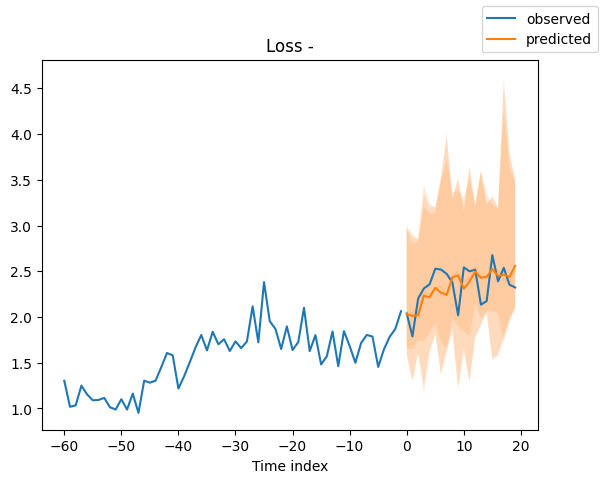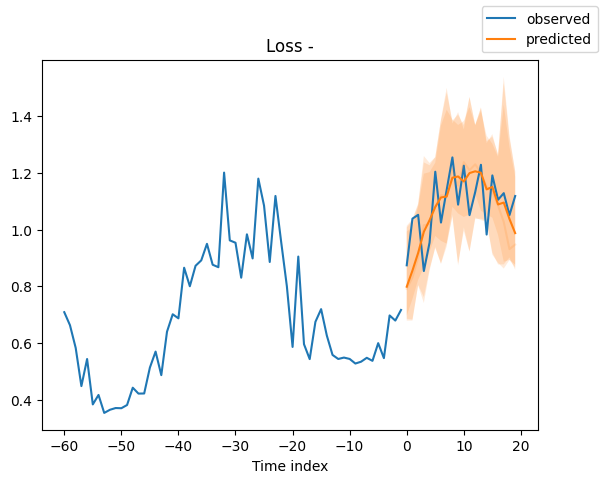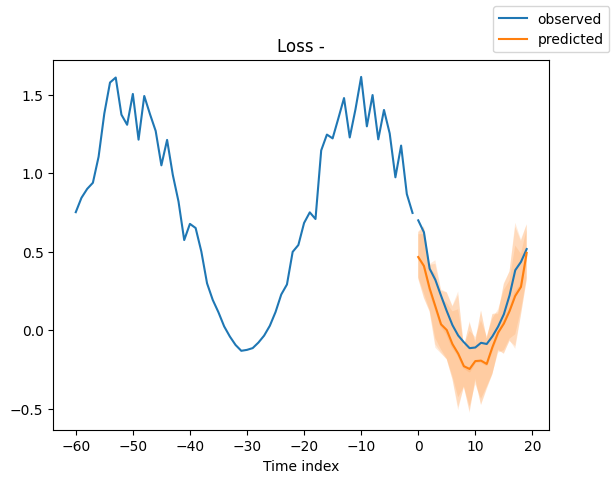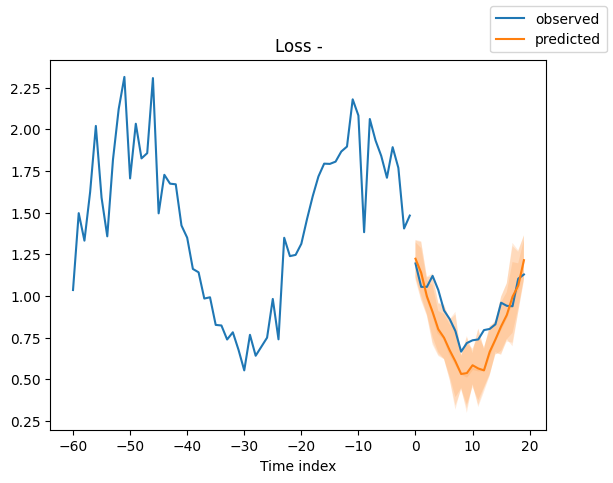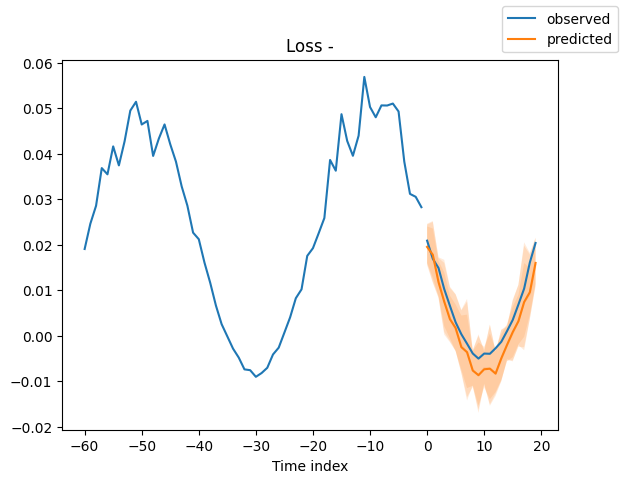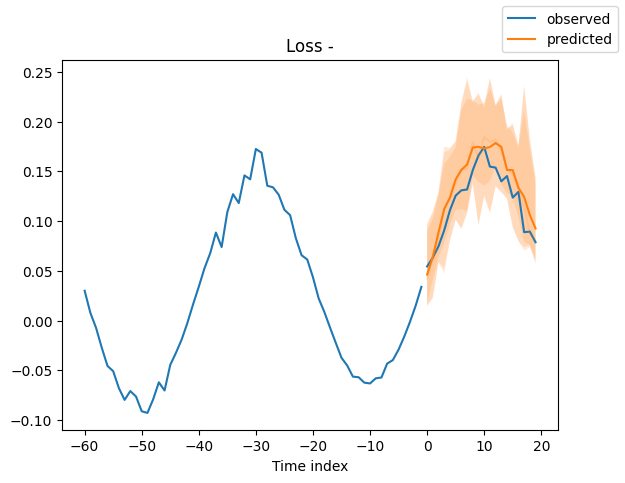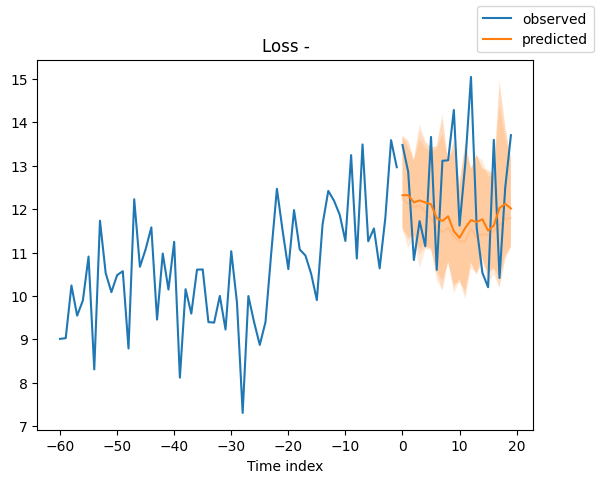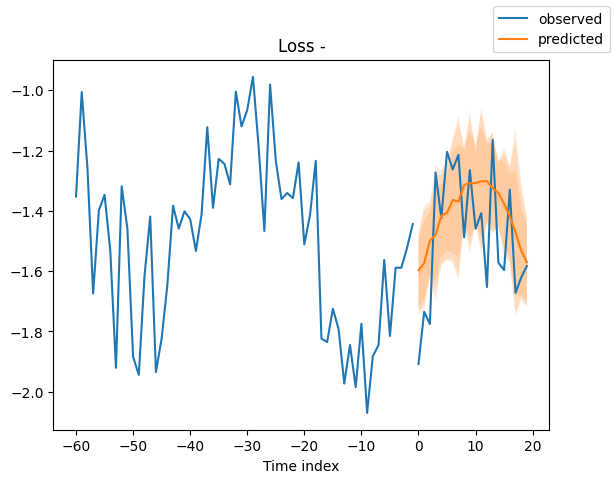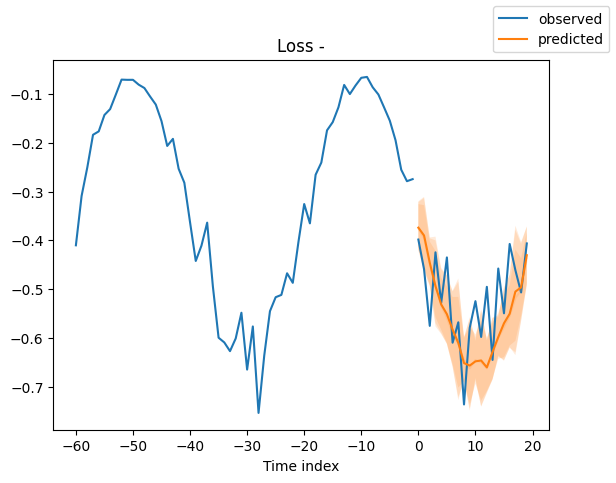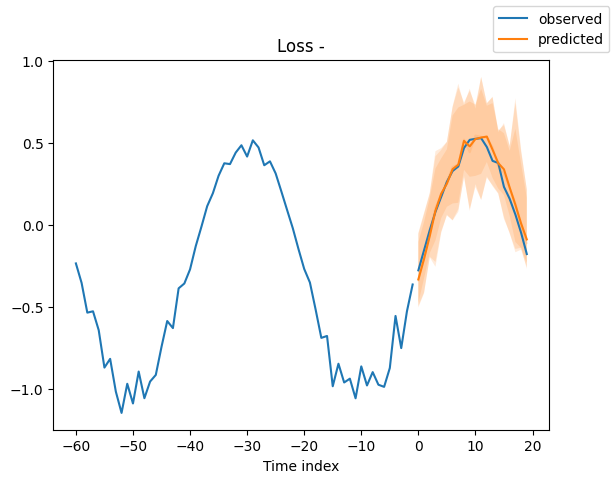## Interpret model#

We can ask PyTorch Forecasting to decompose the prediction into blocks which focus on a different frequency spectrum, e.g. seasonality and trend with plot_interpretation(). This is a special feature of the NHiTS model and only possible because of its unique architecture. The results show that there seem to be many ways to explain the data and the algorithm does not always chooses the one making intuitive sense. This is partially down to the small number of time series we trained on (100). But it is also due because our forecasting period does not cover multiple seasonalities.

:

for idx in range(2):  # plot 10 examples
best_model.plot_interpretation(x, raw_predictions, idx=idx);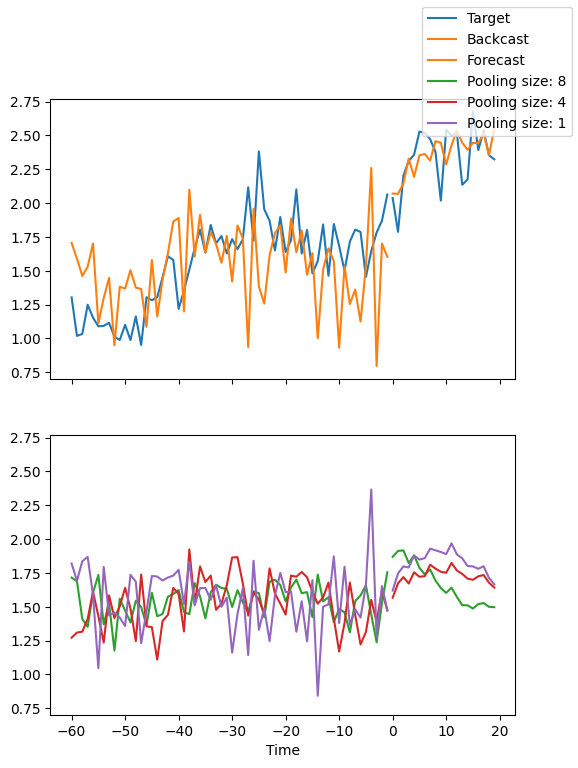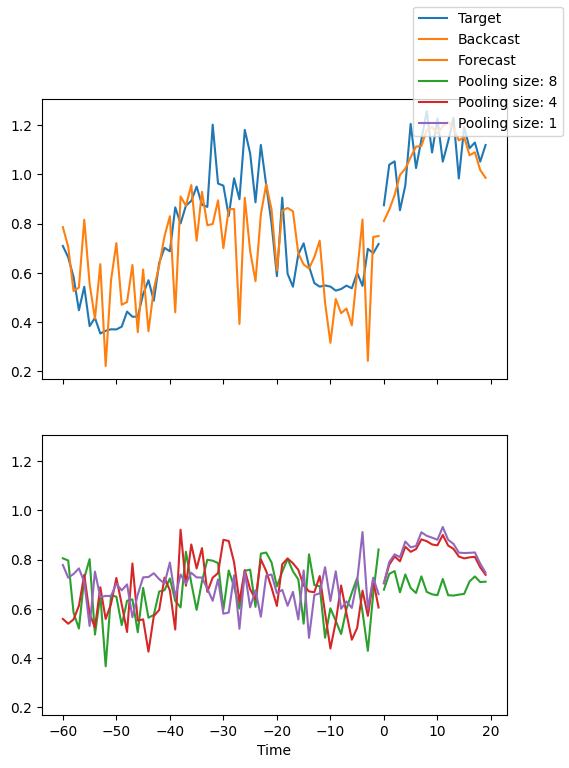## Sampling from predictions#

:

# sample 500 paths
samples = best_model.loss.sample(raw_predictions["prediction"][], n_samples=500)

# plot prediction
fig = best_model.plot_prediction(x, raw_predictions, idx=0, add_loss_to_title=True)
ax = fig.get_axes()
# plot first two sampled paths
ax.plot(samples[:, 0], color="g", label="Sample 1")
ax.plot(samples[:, 1], color="r", label="Sample 2")
fig.legend();As expected, the variance of predictions is smaller within each sample than accross all samples.

:

print(f"Var(all samples) = {samples.var():.4f}")
print(f"Mean(Var(sample)) = {samples.var(0).mean():.4f}")

Var(all samples) = 0.0685
Mean(Var(sample)) = 0.0548


We can now do something new and plot the distribution of sums of forecasts over the entire prediction range.

:

plt.hist(samples.sum(0).numpy(), bins=30)
plt.xlabel("Sum of predictions")
plt.ylabel("Frequency");